# http www comp nus edu sgcs 1010 UNIT

• Slides: 40http: //www. comp. nus. edu. sg/~cs 1010/ UNIT 17 Recursion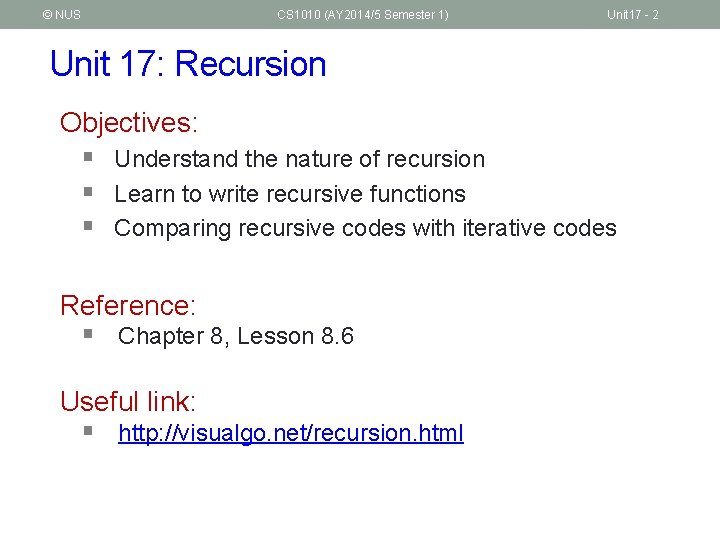© NUS CS 1010 (AY 2014/5 Semester 1) Unit 17 - 2 Unit 17: Recursion Objectives: § Understand the nature of recursion § Learn to write recursive functions § Comparing recursive codes with iterative codes Reference: § Chapter 8, Lesson 8. 6 Useful link: § http: //visualgo. net/recursion. html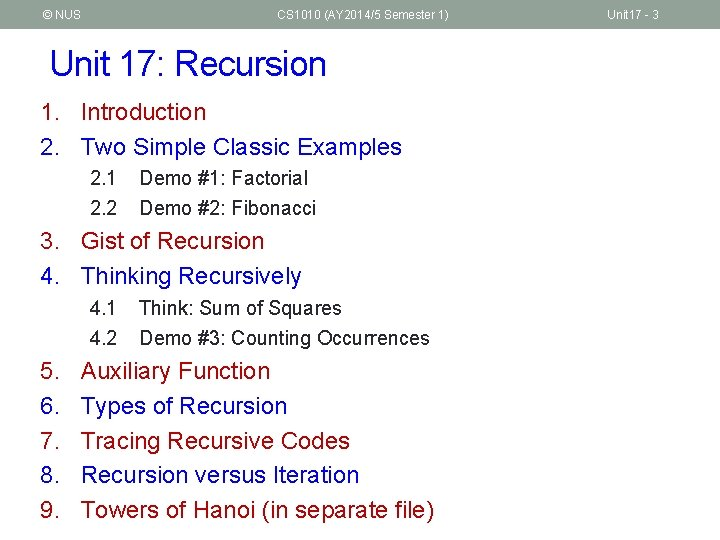© NUS CS 1010 (AY 2014/5 Semester 1) Unit 17: Recursion 1. Introduction 2. Two Simple Classic Examples 2. 1 2. 2 Demo #1: Factorial Demo #2: Fibonacci 3. Gist of Recursion 4. Thinking Recursively 4. 1 4. 2 5. 6. 7. 8. 9. Think: Sum of Squares Demo #3: Counting Occurrences Auxiliary Function Types of Recursion Tracing Recursive Codes Recursion versus Iteration Towers of Hanoi (in separate file) Unit 17 - 3© NUS CS 1010 (AY 2014/5 Semester 1) Unit 17 - 4 1. Introduction (1/3) Some examples of recursion (inside and outside CS): Garfield dreaming recursively. Sierpinksi triangle Droste effect Recursive tree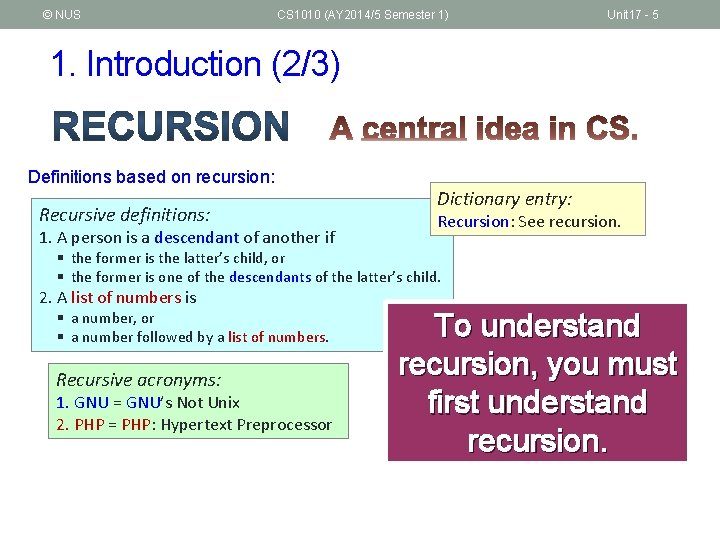© NUS CS 1010 (AY 2014/5 Semester 1) Unit 17 - 5 1. Introduction (2/3) Definitions based on recursion: Recursive definitions: 1. A person is a descendant of another if Dictionary entry: Recursion: See recursion. § the former is the latter’s child, or § the former is one of the descendants of the latter’s child. 2. A list of numbers is § a number, or § a number followed by a list of numbers. Recursive acronyms: 1. GNU = GNU’s Not Unix 2. PHP = PHP: Hypertext Preprocessor To understand recursion, you must first understand recursion.© NUS CS 1010 (AY 2014/5 Semester 1) Unit 17 - 6 1. Introduction (3/3) § There is NO new syntax needed for recursion. § Recursion is a form of (algorithm) design; it is a problemsolving technique for divide-and-conquer paradigm § Very important paradigm – many CS problems solved using it § Recursion is: A method where the solution to a problem depends on solutions to smaller instances of the SAME problem.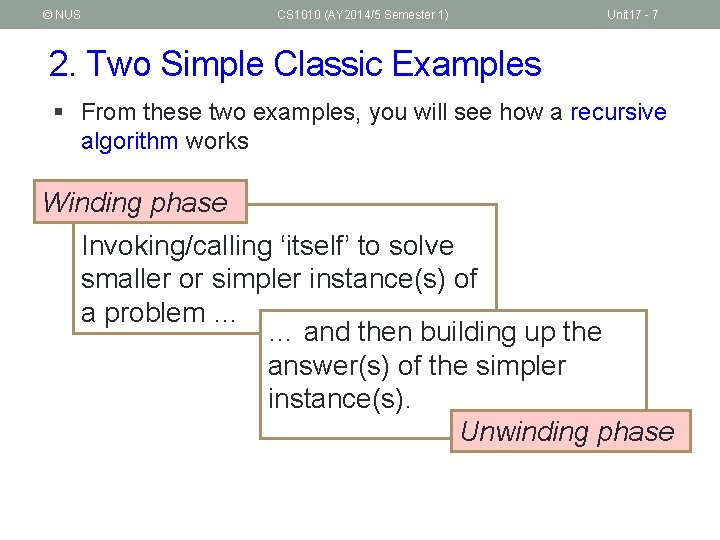© NUS CS 1010 (AY 2014/5 Semester 1) Unit 17 - 7 2. Two Simple Classic Examples § From these two examples, you will see how a recursive algorithm works Winding phase Invoking/calling ‘itself’ to solve smaller or simpler instance(s) of a problem … … and then building up the answer(s) of the simpler instance(s). Unwinding phase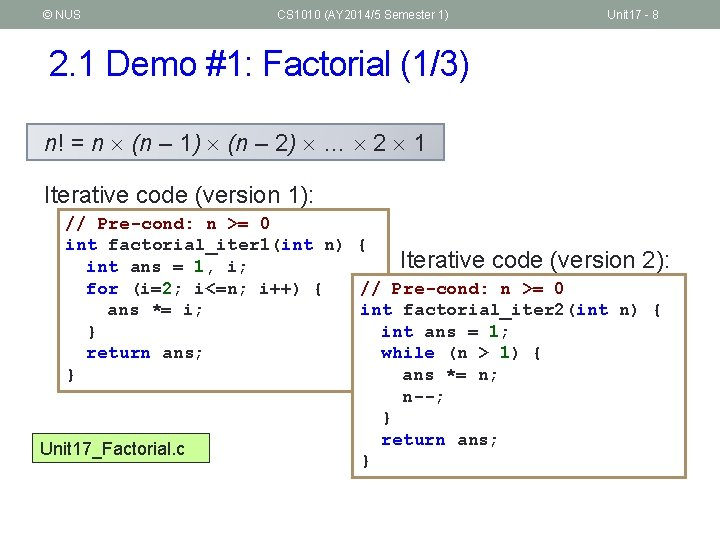© NUS CS 1010 (AY 2014/5 Semester 1) Unit 17 - 8 2. 1 Demo #1: Factorial (1/3) n! = n (n – 1) (n – 2) … 2 1 Iterative code (version 1): // Pre-cond: n >= 0 int factorial_iter 1(int n) { Iterative code (version 2): int ans = 1, i; // Pre-cond: n >= 0 for (i=2; i<=n; i++) { int factorial_iter 2(int n) { ans *= i; int ans = 1; } return ans; while (n > 1) { ans *= n; } n--; } return ans; Unit 17_Factorial. c }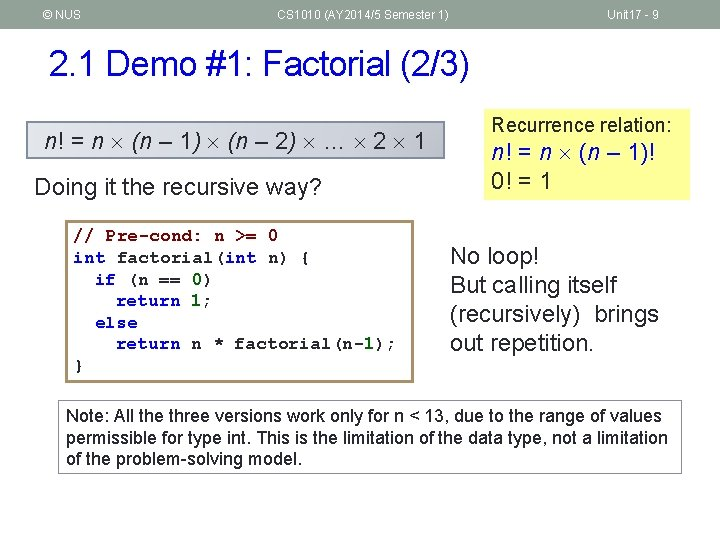© NUS CS 1010 (AY 2014/5 Semester 1) Unit 17 - 9 2. 1 Demo #1: Factorial (2/3) n! = n (n – 1) (n – 2) … 2 1 Doing it the recursive way? // Pre-cond: n >= 0 int factorial(int n) { if (n == 0) return 1; else return n * factorial(n-1); } Recurrence relation: n! = n (n – 1)! 0! = 1 No loop! But calling itself (recursively) brings out repetition. Note: All the three versions work only for n < 13, due to the range of values permissible for type int. This is the limitation of the data type, not a limitation of the problem-solving model.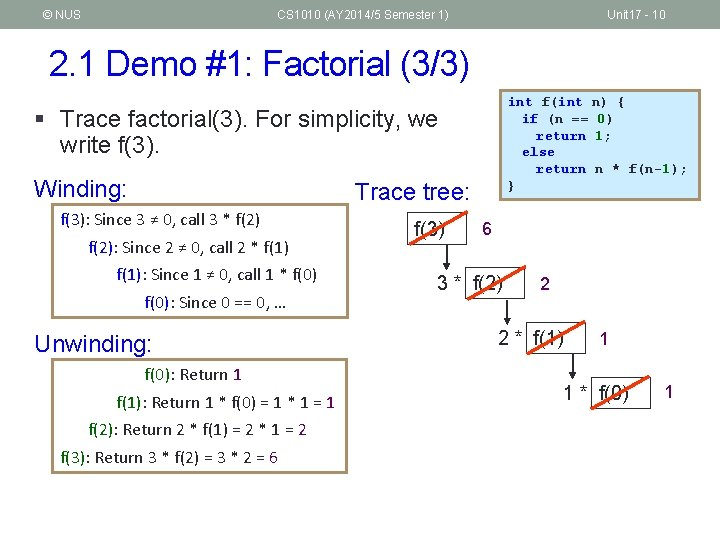© NUS CS 1010 (AY 2014/5 Semester 1) Unit 17 - 10 2. 1 Demo #1: Factorial (3/3) int f(int n) { if (n == 0) return 1; else return n * f(n-1); } § Trace factorial(3). For simplicity, we write f(3). Winding: Trace tree: f(3): Since 3 ≠ 0, call 3 * f(2): Since 2 ≠ 0, call 2 * f(1): Since 1 ≠ 0, call 1 * f(0): Since 0 == 0, … Unwinding: f(0): Return 1 f(1): Return 1 * f(0) = 1 * 1 = 1 f(2): Return 2 * f(1) = 2 * 1 = 2 f(3): Return 3 * f(2) = 3 * 2 = 6 f(3) 6 3 * f(2) 2 2 * f(1) 1 1 * f(0) 1© NUS CS 1010 (AY 2014/5 Semester 1) Unit 17 - 11 2. 2 Demo #2: Fibonacci (1/4) § The Fibonacci series models the rabbit population each time they mate: 1, 1, 2, 3, 5, 8, 13, 21, … § The modern version is: 0, 1, 1, 2, 3, 5, 8, 13, 21, … § Fibonacci numbers are found in nature (sea-shells, sunflowers, etc) § http: //www. maths. surrey. ac. uk/hosted-sites/R. Knott/Fibonacci/fibnat. html© NUS CS 1010 (AY 2014/5 Semester 1) Unit 17 - 12 2. 2 Demo #2: Fibonacci (2/4) § Fibonacci numbers are found in nature (sea-shells, sunflowers, etc) § http: //www. maths. surrey. ac. uk/hostedsites/R. Knott/Fibonacci/fibnat. html© NUS CS 1010 (AY 2014/5 Semester 1) Unit 17 - 13 2. 2 Demo #2: Fibonacci (3/4) 0, 1, 1, 2, 3, 5, 8, 13, 21, … Iterative code: // Pre-cond: n >= 0 int fib_iter(int n) { int prev 1 = 1, prev 2 = 0, sum; if (n < 2) return n; for (; n>1; n--) { sum = prev 1 + prev 2; prev 2 = prev 1; prev 1 = sum; } return sum; } Unit 17_Fibonacci. c Recursive code: // Pre-cond: n >= 0 int fib(int n) { if (n < 2) return n; else return fib(n-1) + fib(n-2); } Recurrence relation: fn = fn-1 + fn-2 n ≥ 2 f 0 = 0 f 1 = 1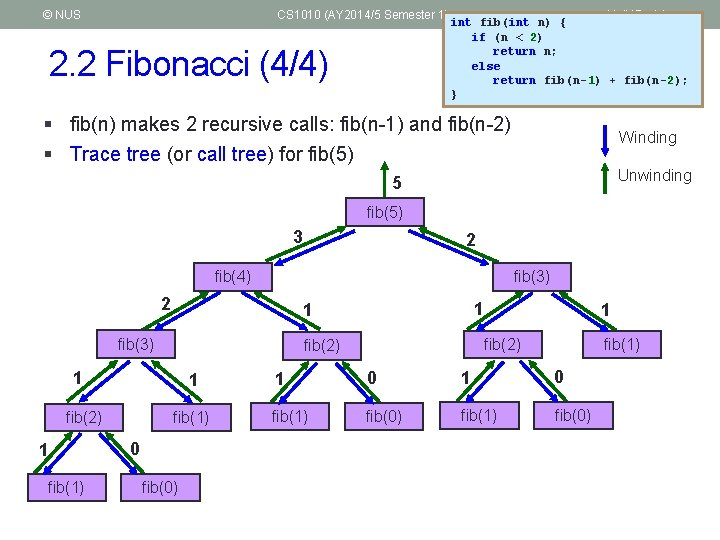© NUS CS 1010 (AY 2014/5 Semester 1) 2. 2 Fibonacci (4/4) Unit 17 - 14 int fib(int n) { if (n < 2) return n; else return fib(n-1) + fib(n-2); } § fib(n) makes 2 recursive calls: fib(n-1) and fib(n-2) § Trace tree (or call tree) for fib(5) Winding Unwinding 5 fib(5) 3 2 fib(4) fib(3) 2 fib(3) 1 1 fib(1) 0 fib(0) 1 fib(2) 1 1 fib(1) 1 0 fib(1) fib(0)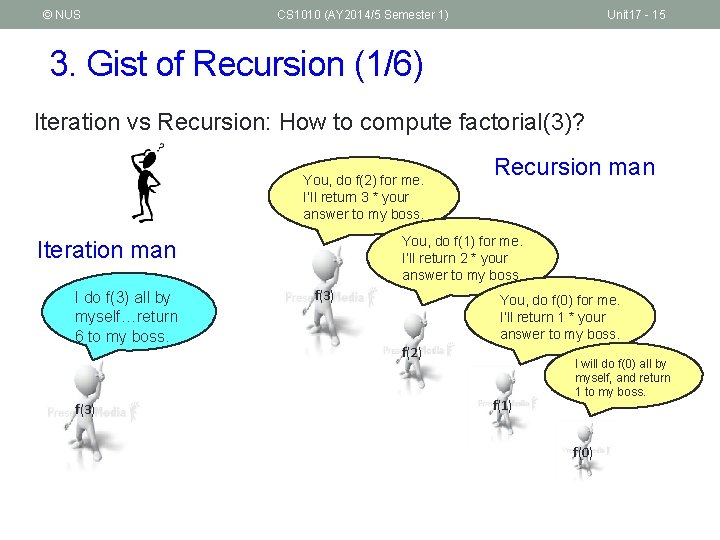© NUS CS 1010 (AY 2014/5 Semester 1) Unit 17 - 15 3. Gist of Recursion (1/6) Iteration vs Recursion: How to compute factorial(3)? You, do f(2) for me. I’ll return 3 * your answer to my boss. You, do f(1) for me. I’ll return 2 * your answer to my boss. Iteration man I do f(3) all by myself…return 6 to my boss. f(3) Recursion man f(3) You, do f(0) for me. I’ll return 1 * your answer to my boss. f(2) f(1) I will do f(0) all by myself, and return 1 to my boss. f(0)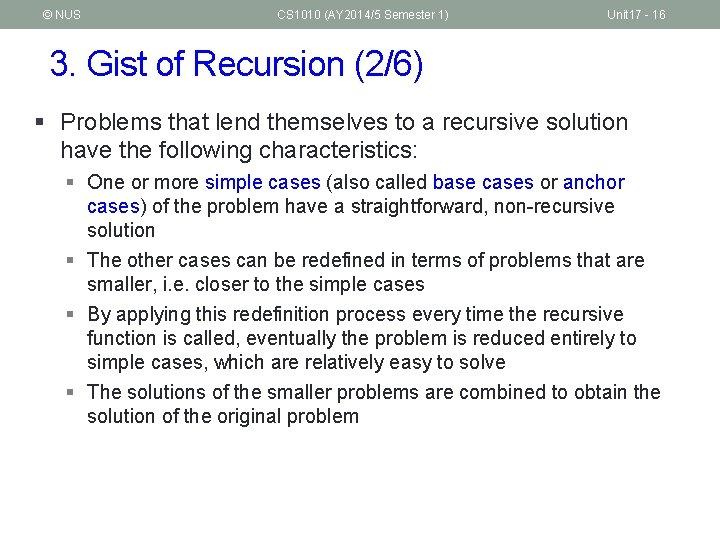© NUS CS 1010 (AY 2014/5 Semester 1) Unit 17 - 16 3. Gist of Recursion (2/6) § Problems that lend themselves to a recursive solution have the following characteristics: § One or more simple cases (also called base cases or anchor cases) of the problem have a straightforward, non-recursive solution § The other cases can be redefined in terms of problems that are smaller, i. e. closer to the simple cases § By applying this redefinition process every time the recursive function is called, eventually the problem is reduced entirely to simple cases, which are relatively easy to solve § The solutions of the smaller problems are combined to obtain the solution of the original problem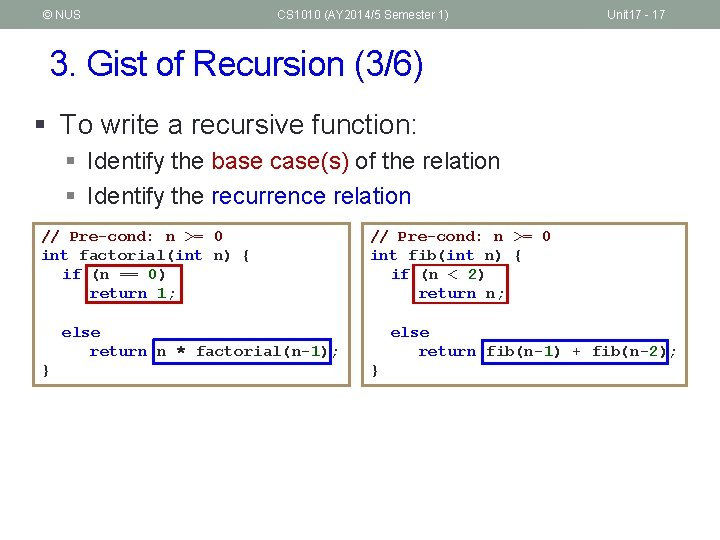© NUS CS 1010 (AY 2014/5 Semester 1) Unit 17 - 17 3. Gist of Recursion (3/6) § To write a recursive function: § Identify the base case(s) of the relation § Identify the recurrence relation // Pre-cond: n >= 0 int factorial(int n) { if (n == 0) return 1; // Pre-cond: n >= 0 int fib(int n) { if (n < 2) return n; else return n * factorial(n-1); } else return fib(n-1) + fib(n-2); }© NUS CS 1010 (AY 2014/5 Semester 1) 3. Gist of Recursion (4/6) § Always check for base case(s) first § What if you omit base case(s)? § Do not write redundant base cases int factorial(int n) { if (n == 0) return 1; else if (n == 1) return 1; else if (n == 2) redundant return 2; else if (n == 3) return 6; else return n * factorial(n-1); } Unit 17 - 18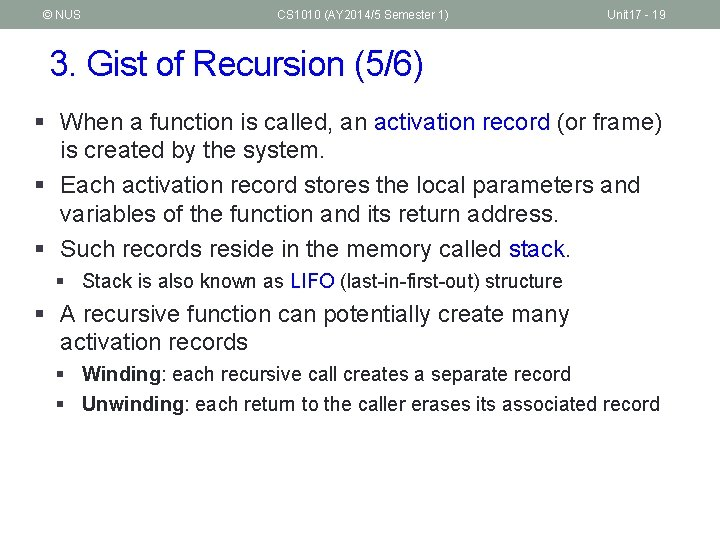© NUS CS 1010 (AY 2014/5 Semester 1) Unit 17 - 19 3. Gist of Recursion (5/6) § When a function is called, an activation record (or frame) is created by the system. § Each activation record stores the local parameters and variables of the function and its return address. § Such records reside in the memory called stack. § Stack is also known as LIFO (last-in-first-out) structure § A recursive function can potentially create many activation records § Winding: each recursive call creates a separate record § Unwinding: each return to the caller erases its associated record© NUS CS 1010 (AY 2014/5 Semester 1) 3. Gist of Recursion (6/6) § Example: factorial(3) n n 3 f(3) n 2 3 f(2) n n 1 2 3 f(1) n n n Unit 17 - 20 int f(int n) { if (n == 0) return 1; else return n * f(n-1); } 0 1 1 n 2 n 3 f(0) n n 3 6 1 1 2 3 2 2 3© NUS CS 1010 (AY 2014/5 Semester 1) 4. Thinking Recursively § It is apparent that to do recursion you need to think “recursively”: § Breaking a problem into simpler problems that have identical form § Is there only one way of breaking a problem into simpler problems? Unit 17 - 21© NUS CS 1010 (AY 2014/5 Semester 1) Unit 17 - 22 4. 1 Think: Sum of Squares (1/5) § Given 2 positive integers x and y, where x y, compute sum. Sq(x, y) = x 2 + (x+1) 2 + … + (y-1) 2 + y 2 • For example sum. Sq(5, 10) = 52 + 62 + 72 + 82 + 92 + 102 = 355 § How do you break this problem into smaller problems? § How many ways can it be done? § We are going to show 3 versions § See Unit 17_Sum. Squares. c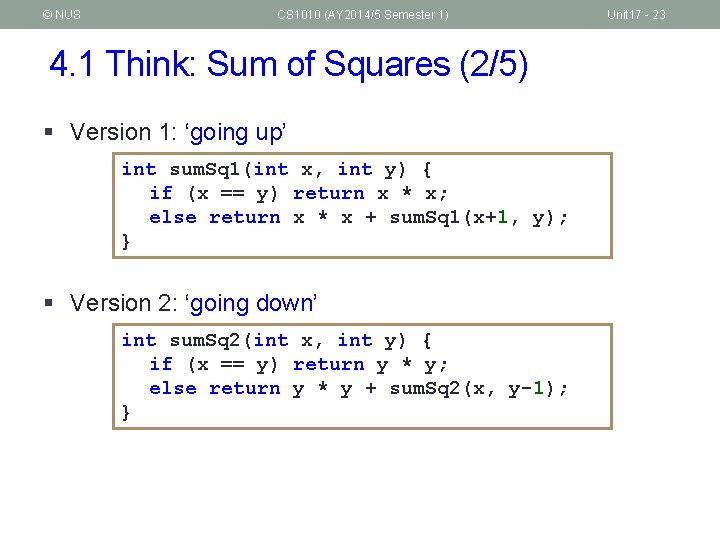© NUS CS 1010 (AY 2014/5 Semester 1) 4. 1 Think: Sum of Squares (2/5) § Version 1: ‘going up’ int sum. Sq 1(int x, int y) { if (x == y) return x * x; else return x * x + sum. Sq 1(x+1, y); } § Version 2: ‘going down’ int sum. Sq 2(int x, int y) { if (x == y) return y * y; else return y * y + sum. Sq 2(x, y-1); } Unit 17 - 23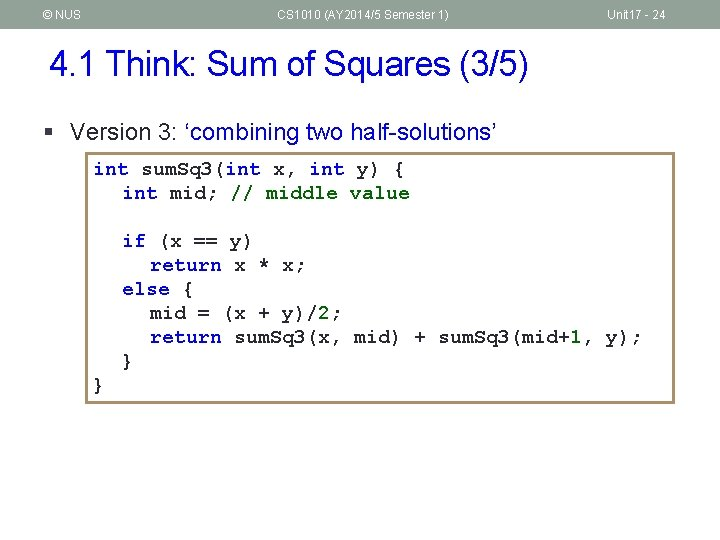© NUS CS 1010 (AY 2014/5 Semester 1) Unit 17 - 24 4. 1 Think: Sum of Squares (3/5) § Version 3: ‘combining two half-solutions’ int sum. Sq 3(int x, int y) { int mid; // middle value if (x == y) return x * x; else { mid = (x + y)/2; return sum. Sq 3(x, mid) + sum. Sq 3(mid+1, y); } }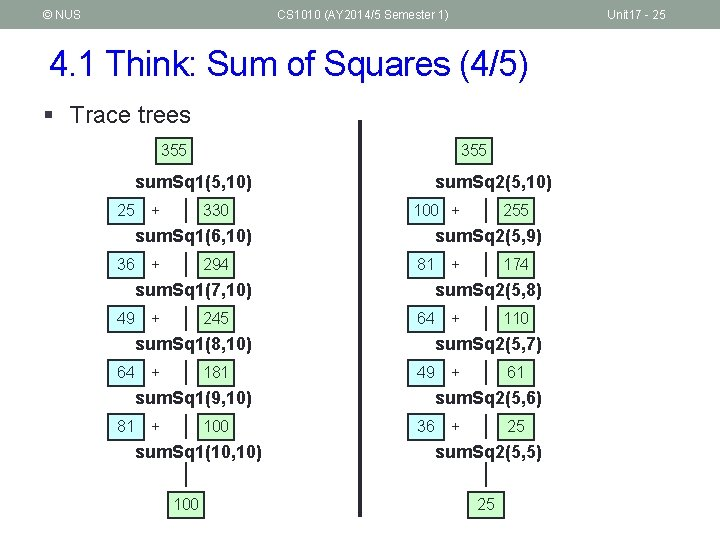© NUS CS 1010 (AY 2014/5 Semester 1) Unit 17 - 25 4. 1 Think: Sum of Squares (4/5) § Trace trees 355 sum. Sq 1(5, 10) 25 + 330 sum. Sq 1(6, 10) 36 + 294 sum. Sq 1(7, 10) 49 + 245 sum. Sq 1(8, 10) 64 + 181 sum. Sq 1(9, 10) 81 + 100 sum. Sq 1(10, 10) 100 sum. Sq 2(5, 10) 100 + 255 sum. Sq 2(5, 9) 81 + 174 sum. Sq 2(5, 8) 64 + 110 sum. Sq 2(5, 7) 49 + 61 sum. Sq 2(5, 6) 36 25 + sum. Sq 2(5, 5) 25© NUS CS 1010 (AY 2014/5 Semester 1) Unit 17 - 26 4. 1 Think: Sum of Squares (5/5) § Trace tree 355 sum. Sq 3(5, 10) 110 245 sum. Sq 3(5, 7) sum. Sq 3(8, 10) 61 49 sum. Sq 3(5, 6) 25 sum. Sq 3(7, 7) 36 sum. Sq 3(5, 5) 25 145 sum. Sq 3(6, 6) 36 49 100 sum. Sq 3(8, 9) 64 sum. Sq 3(10, 10) 81 sum. Sq 3(8, 8) 64 sum. Sq 3(9, 9) 81 100© NUS CS 1010 (AY 2014/5 Semester 1) Unit 17 - 27 4. 2 Demo #3: Counting Occurrences (1/4) § Given an array int list[ ] = { 9, -2, 1, 7, 3, 9, -5, 7, 2, 1, 7, -2, 0, 8, -3 } § We want count. Value(7, list, 15) to return 3 (the number of times 7 appears in the 15 elements of list.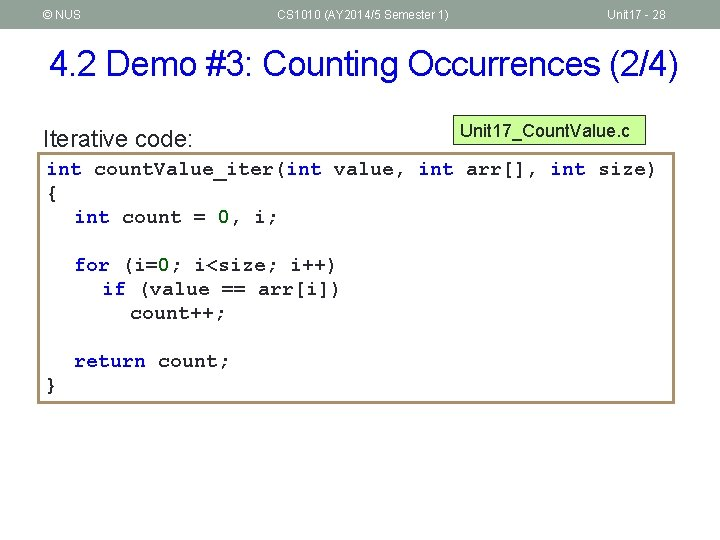© NUS CS 1010 (AY 2014/5 Semester 1) Unit 17 - 28 4. 2 Demo #3: Counting Occurrences (2/4) Iterative code: Unit 17_Count. Value. c int count. Value_iter(int value, int arr[], int size) { int count = 0, i; for (i=0; i<size; i++) if (value == arr[i]) count++; return count; }© NUS CS 1010 (AY 2014/5 Semester 1) Unit 17 - 29 4. 2 Demo #3: Counting Occurrences (3/4) § To get count. Value(7, list, 15) to return 3. § Recursive thinking goes… 9 -2 1 7 3 9 -5 7 2 1 7 … and get someone to count the 7 in this smaller problem, … -2 0 8 -3 If I handle the last element myself, … … then, depending on whether the last element is 7 or not, my answer is either his answer or his answer plus 1!© NUS CS 1010 (AY 2014/5 Semester 1) Unit 17 - 30 4. 2 Demo #3: Counting Occurrences (4/4) Recursive code: Unit 17_Count. Value. c int count. Value(int value, int arr[], int size) { if (size == 0) return 0; else return (value == arr[size-1]) + count. Value(value, arr, size-1); } Note: The second return statement is equivalent to the following (why? ): if (value == arr[size-1]) return 1 + count. Value(value, arr, size-1); else return count. Value(value, arr, size-1);© NUS CS 1010 (AY 2014/5 Semester 1) Unit 17 - 31 5. Auxiliary Function (1/3) § Sometimes, auxiliary functions are needed to implement recursion. Eg: Refer to Demo #3 Counting Occurrences. § If the function handles the first element instead of the last, it could be re-written as follows: int count. Value(int value, int arr[], int start, int size) { if (start == size) return 0; else return (value == arr[start]) + count. Value(value, arr, start+1, size); }© NUS CS 1010 (AY 2014/5 Semester 1) Unit 17 - 32 5. Auxiliary Function (2/3) § However, doing so means that the calling function has to change the call from: count. Value(value, list, ARRAY_SIZE) to: count. Value(value, list, 0, ARRAY_SIZE) § The additional parameter 0 seems like a redundant data from the caller’s point of view.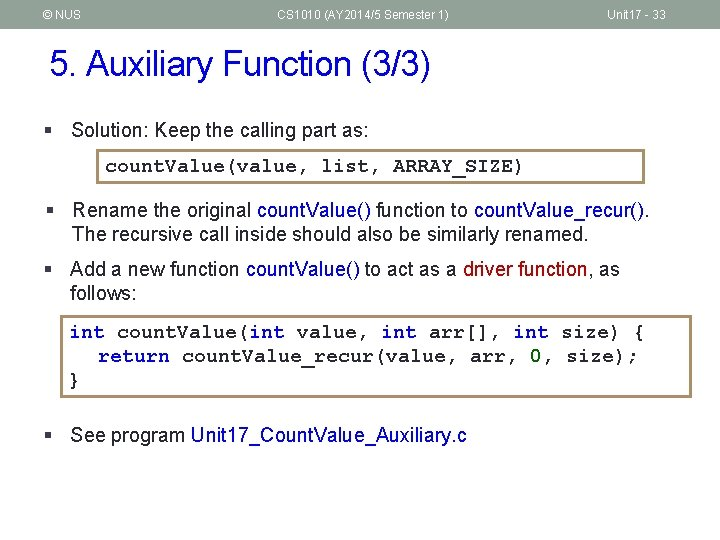© NUS CS 1010 (AY 2014/5 Semester 1) Unit 17 - 33 5. Auxiliary Function (3/3) § Solution: Keep the calling part as: count. Value(value, list, ARRAY_SIZE) § Rename the original count. Value() function to count. Value_recur(). The recursive call inside should also be similarly renamed. § Add a new function count. Value() to act as a driver function, as follows: int count. Value(int value, int arr[], int size) { return count. Value_recur(value, arr, 0, size); } § See program Unit 17_Count. Value_Auxiliary. c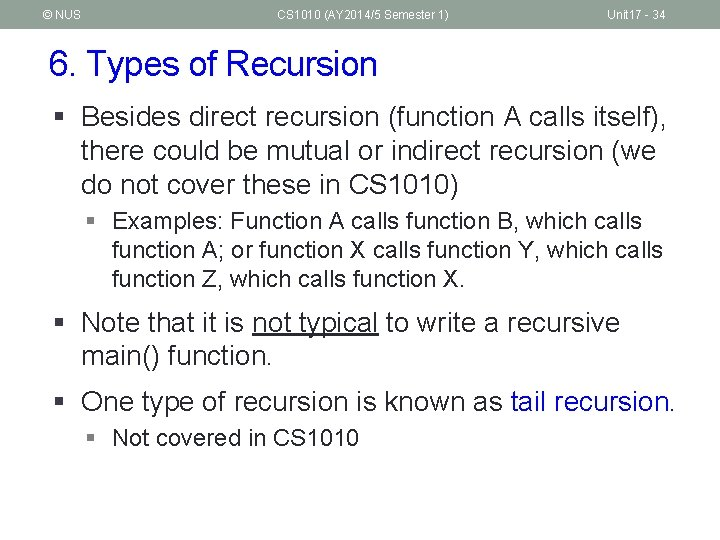© NUS CS 1010 (AY 2014/5 Semester 1) Unit 17 - 34 6. Types of Recursion § Besides direct recursion (function A calls itself), there could be mutual or indirect recursion (we do not cover these in CS 1010) § Examples: Function A calls function B, which calls function A; or function X calls function Y, which calls function Z, which calls function X. § Note that it is not typical to write a recursive main() function. § One type of recursion is known as tail recursion. § Not covered in CS 1010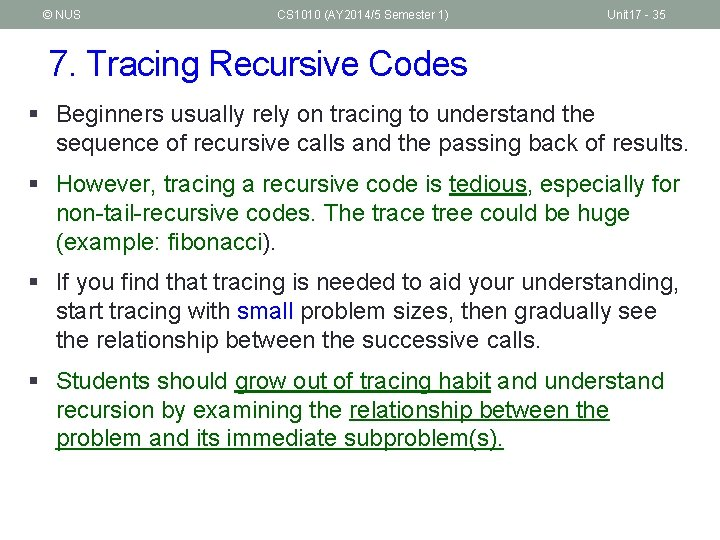© NUS CS 1010 (AY 2014/5 Semester 1) Unit 17 - 35 7. Tracing Recursive Codes § Beginners usually rely on tracing to understand the sequence of recursive calls and the passing back of results. § However, tracing a recursive code is tedious, especially for non-tail-recursive codes. The trace tree could be huge (example: fibonacci). § If you find that tracing is needed to aid your understanding, start tracing with small problem sizes, then gradually see the relationship between the successive calls. § Students should grow out of tracing habit and understand recursion by examining the relationship between the problem and its immediate subproblem(s).© NUS CS 1010 (AY 2014/5 Semester 1) Unit 17 - 36 8. Recursion versus Iteration (1/2) § Iteration can be more efficient § Replaces function calls with looping § Less memory is used (no activation record for each call) § Some good compilers are able to transform a tail-recursion code into an iterative code. § General guideline: If a problem can be done easily with iteration, then do it with iteration. § For example, Fibonacci can be coded with iteration or recursion, but the recursive version is very inefficient (large call tree due to duplicate computations), so use iteration instead.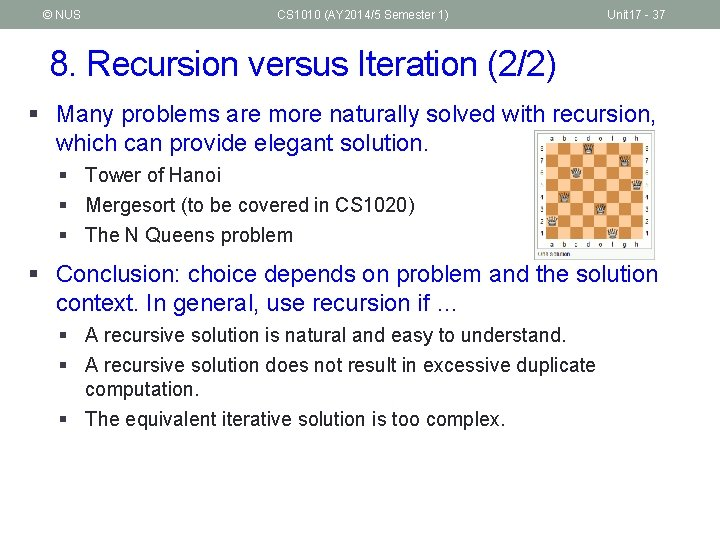© NUS CS 1010 (AY 2014/5 Semester 1) Unit 17 - 37 8. Recursion versus Iteration (2/2) § Many problems are more naturally solved with recursion, which can provide elegant solution. § Tower of Hanoi § Mergesort (to be covered in CS 1020) § The N Queens problem § Conclusion: choice depends on problem and the solution context. In general, use recursion if … § A recursive solution is natural and easy to understand. § A recursive solution does not result in excessive duplicate computation. § The equivalent iterative solution is too complex.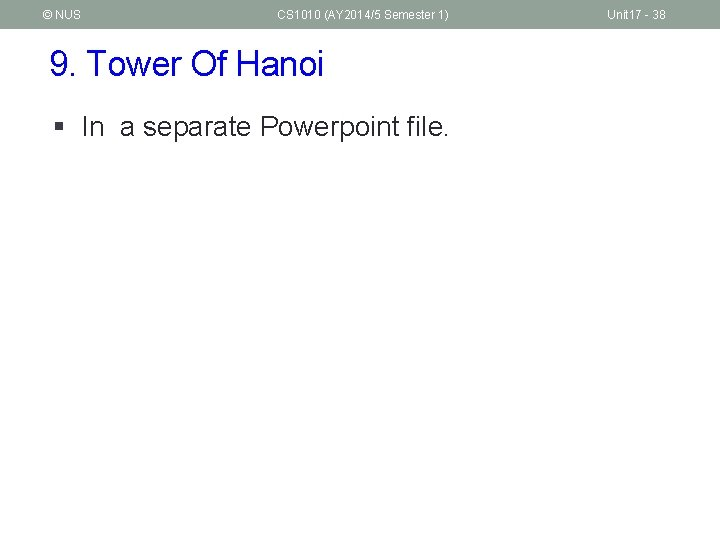© NUS CS 1010 (AY 2014/5 Semester 1) 9. Tower Of Hanoi § In a separate Powerpoint file. Unit 17 - 38© NUS CS 1010 (AY 2014/5 Semester 1) Summary n In this unit, you have learned about n Recursion as a design strategy n The components of a recursive code n Differences between Recursion and Iteration Unit 17 - 39© NUS CS 1010 (AY 2014/5 Semester 1) End of File Unit 17 - 40